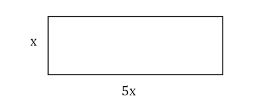# The rectangle below has an area of 245 $$\text{inches} ^2$$. What is the length of the longest side of the rectangle?35 in

Explanation

A rectangle's area is length times width. Here, length is 5x and width is x so:

$$\begin{gathered} 245 \mathrm{in}^2=x \times 5 x \\ 245 \text { in }^2=5 x^2 \\ 49 \text { in }^2=x^2 \\ 7 \text { in }=x \end{gathered}$$

Therefore, the longest side is $$5 \times 7 \text { in }=35 \mathrm{in}$$